### Step by Step Rubik's Cube

RUBIK’S CUBE 3×3×3
Rubik’s cube is the most challenging trick to solve it. If you can get all side in the Rubik's cube contain the same color, you will be satisfied. Otherwise, you will be disappointed and give up trying it again. If you are still motivated to do this challenge, I will reveal to you the entire step on how to get it done. The first thing that you should know is the name of the small cube.

Center = cube that static in the middle of the Rubik's cube. They are 6 center in the Rubik's cube which is center yellow, center white, center green, center blue, center red, and center orange.
Edge = Each side of the Rubik's cube contains 4 edges. Edge contain 2 colour(2 side) only.
Corner edge = Each side of the Rubik's cube contain 4 corner edge. Corner edge contains 3 colors (3 sides) on their surface

Figure 1 below shows the position of center, edge, and corner edge.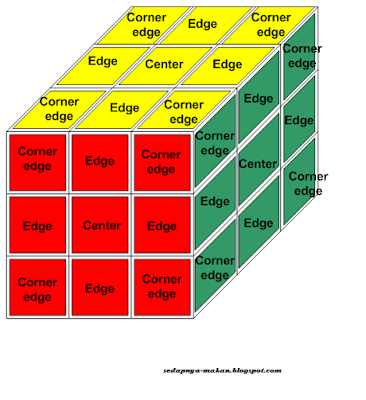Figure 1: center, edge, and corner edge.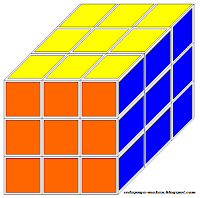Figure 2 : Rubik's Cube color

Before we can solve the Rubik’s cube, there is some basic knowledge that we should know.  We need to know the color on each side of the cube. The color involves in this cube are RED, YELLOW, BLUE, ORANGE, WHITE and GREEN as shown in figure 2.
Center GREEN is always opposite with center BLUE
Center WHITE is always opposite with center YELLOW
Center RED is always opposite with center ORANGE

MOVEMENT
In order to solve Rubik's cube, there are some moves that we should know. To be an expert player, you should memories all the moving steps. In this case, to move one cube whether corner edge or edge to the right position, we need several steps to complete the task. The combination of all steps is called an algorithm. These algorithms are fixed for some cases. All you need to know is, if each case occurs at the moment of solving the Rubik's cube, you should know which algorithm that can be used.
Before we discuss further on the algorithm, let’s see the basic movement of the Rubik's cube and what are the key’s or formula or name’s to memorize all of them.

PATTERN OF RUBIK’S CUBE
To complete the task, they are several steps that we need to follow. Below is the Rubik's cube pattern start from the beginning until we solve the entire Rubik's cube.

1. Get the WHITE cross on top. The EDGE color must be the same as CENTER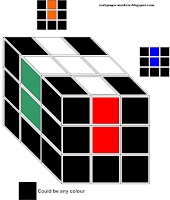Figure 3: Rubik’s cube pattern 1

2. Get all WHITE color in position (we solved WHITE color side). CORNER EDGE at WHITE side must be in color with other two nearest sides.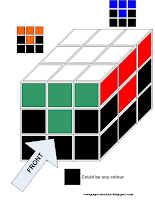Figure 4: Rubik’s cube pattern 2

3. The next step to get the pattern as shown below.Figure 5: Rubik’s cube pattern 3

4. After that, we need to form a YELLOW cross on the top of the Rubik's cube.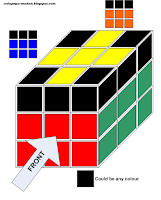Figure 6: Rubik’s cube pattern 4
5.      When finish getting a YELLOW cross, the next task is to complete the YELLOW side. Mean that all the top side must be in YELLOW color.Figure 7: Rubik’s cube pattern 5

6. We are almost close to the target now. The next task is to get Rubik's cube pattern as below.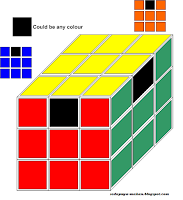Figure 8: Rubik’s cube pattern 6

7. This is the final step where we are able to complete the entire task and make all side are organized with the same color.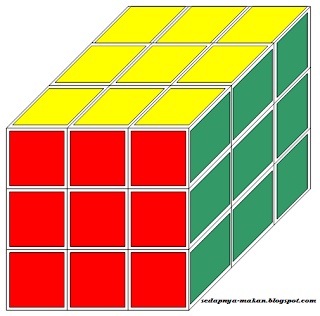Figure 9: Rubik’s cube pattern 7
There are 7 steps that need to be considered when you trying to solve this Rubik's cube. You must follow the entire pattern in 7 steps without fail. Fail to follow any of the steps, you will never found the final stage. The next topic will explain in detail step by step each of them.

STEP 1 (solving pattern 1)
The objective of step 1 is to form a pattern as show in figure 10.  Ignore others colour apart from the WHITE cross, WHITE EDGE and CENTER side colour. The ignore colour’s part are temporary painted with black’s colour. It easy for us to focus which colour is inline with the pattern. Note that we will use black’s colour as ‘don’t care’ colour for the entire steps.figure 10: pattern 1
Basically, for pattern 1, it easy for us to form it. The first step is to make sure the CENTER WHITE is in top / upper positions while the CENTER YELLOW in the down position. Figure 11 shows the position of the CENTER WHITE.Figure 11: center white in the top / upper position
The next step is to make EDGE WHITE in position so that we can form a WHITE cross. The side EDGE must be in line with the CENTER color as shown in figure 12.Figure 12: the position of EDGE WHITE and EDGE side
In some cases, we will face difficulty to arrange between EDGE WHITE and EDGE side as shown in figure 13. In order to solve the problem, we will use algorithm FU’RU (refer table 1 for each movement) as shown in figure 14.Figure 13: EDGE WHITE and EDGE side (for this case EDGE RED) is in reverse position.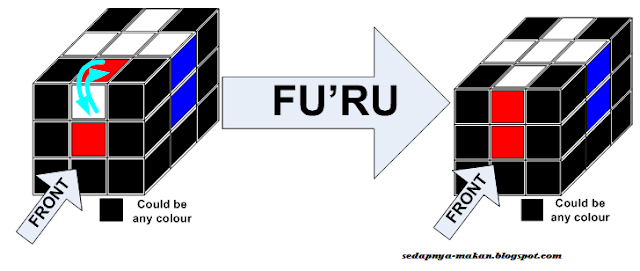Figure 14: Using algorithm FU’RU

STEP 2 (solving pattern 2)
Good job! You have done the task for step 1. Let move on to step 2 which is to solve until we get the pattern as shown in figure 15.figure 15: pattern 2
Step 2 is continuous from the result of step 1 (pattern 1). All we need to do is to complete the CORNER EDGE WHITE and place it in the right position. You must also consider others 2 side color at the CORNER EDGE WHITE. They must be in line with the side color respectively as shown in figure 16. It applied to all 4 CORNER EDGE WHITE on the top/upper.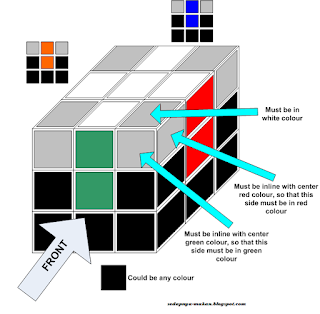Figure 16: the position of CORNER WHITE
In order to solve this pattern, they are several steps and cases need to be considered. For the first step to solve, we bring the CENTER YELLOW to the top/upper. At the top/upper layer, find the white color position. Our guideline to solve is depending on the white color position. Figure 17 shown the illustration of the arrangement.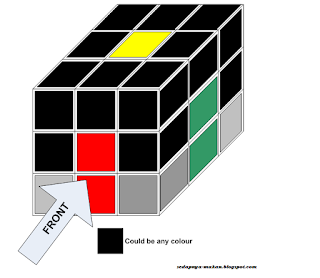Figure 17: CENTER YELLOW to the top/upper
They are several cases involve in order to solve this pattern. The first case is when we find the CORNER EDGE WHITE placing the white color at the right side of the cube. Then we bring the CORNER EDGE WHITE and placed it in between 2 other color that contains at the CORNER EDGE WHITE. The illustration of the arrangement is shown in figure 18.

a) Case 1 (CORNER EDGE WHITE cube = white color placing at the right side)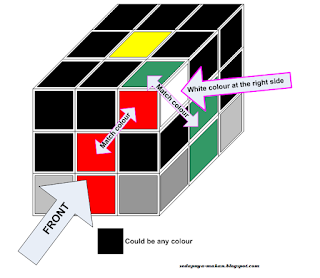Figure 18: Arrangement of case 1 (white color placing at the right side)
For this case, we have to bring that particular cube from top to down so that it will place in the correct position. For that reason, the algorithm used is RUR’. Figure 19 shows the step of using this algorithm.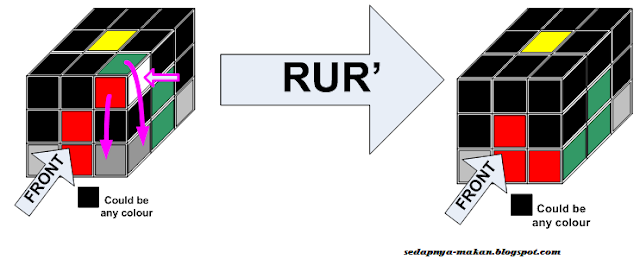Figure 19: Using algorithm RUR’

b) Case 2 (CORNER EDGE WHITE cube = white color placing at the front side)Figure 20: Arrangement of case 2 (white color placing on the front side)
For case 2, CORNER EDGE WHITE cube again are placing at the front right top as in case 1, but white color now is placing at the front. The other two colors must in line with the center color of the right side and front side respectively. Figure 20 shows the arrangement of case 2 while figure 21 shows the algorithm to solve it.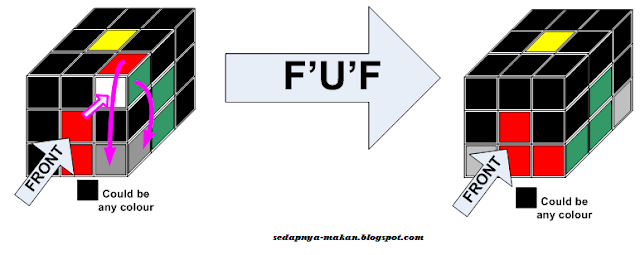Figure 21: Using algorithm F’U’F
c) Case 3 (CORNER EDGE WHITE cube = white color placing at the top/upper side)Figure 22: Arrangement of case 3 (white color placing at the top/upper side)
For case 3, the CORNER EDGE WHITE cube now is placing the white color at the top/upper of the Rubik's cube. We can see the arrangement of case 3 in figure 22. Figure 23 shows the algorithm that can be used to solve the trick.Figure 23: Using algorithm (R U2 R’) U’ (RUR’)

d) Case 4 (CORNER EDGE WHITE cube = white color placing at the bottom of the right side)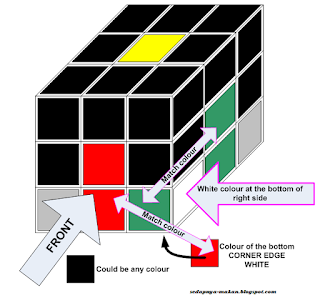Figure 24: Arrangement of case 4 (white color at the bottom of the right side)
For case 4, the CORNER EDGE WHITE is in place at the correct position, but the color is not in the correct position. Figure 24 shows the arrangement of case 4. Figure 25 shows the algorithm used to place the color in the correct position.Figure 25: Using algorithm (RUR’) U’ (RUR’)
e) Case 5 (CORNER EDGE WHITE cube = white color placing at the bottom of front side)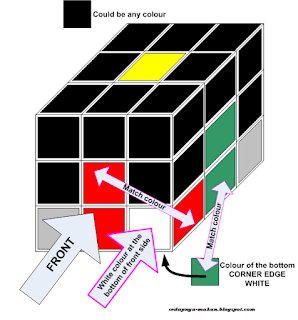Figure 26: Arrangement of case 5 (white color at the bottom of the front side)
Case 5 same as case 4 which is the CORNER EDGE WHITE is in place at the correct position, but the color is not in the correct position. Figure 26 shows the arrangement of case 5. Figure 27 shows the algorithm used to place the color in the correct position.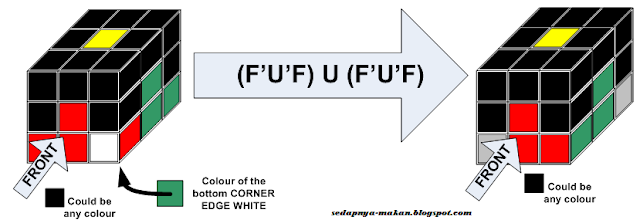Figure 27: Using algorithm (F’U’F) U (F’U’F)

STEP 3 (solving pattern 3)
After finish to solve step 1 and step 2, the next step is to solve pattern 3 in step 3. Illustration of pattern 3 is shown in figure 28. The objective of step 3 is to fulfill the position EDGE cube mark by grey color to the correct color position as shown in figure 29.Figure 28: Rubik's cube pattern 3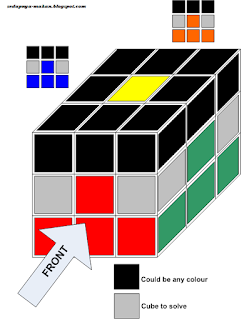Figure 29: Grey cube is the target cube to solve
For the beginning of the step, again the yellow position must be at the top/upper position. At the upper layer, find the EDGE cube which does not contain yellow color. For this case, ignore the yellow color first. There is 3 possible cases in order to solve pattern 3.

a) Case 1 (bring the EDGE cube to the FRONT right side)
Figure 30 is the arrangement position of case 1 and figure 31 show the algorithm involve to solve the position.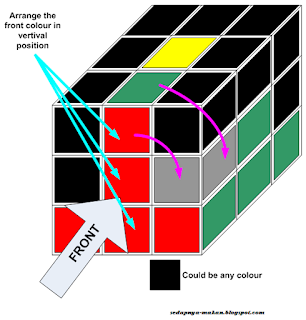Figure 30: Bring FRONT EDGE upper to the FRONT right
dfdfdfd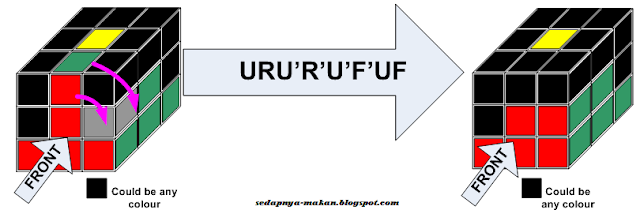Figure 31: using the algorithm URU’R’U’F’UF

b) Case 2 (bring the EDGE cube to the FRONT left side)
Figure 32 is the arrangement position of case 2 and figure 33 show the algorithm involve to solve the position.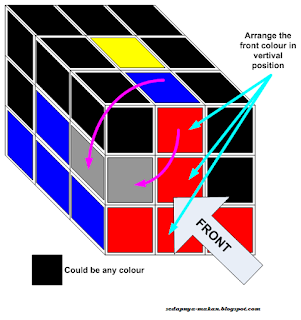Figure 32: Bring FRONT EDGE upper to the FRONT left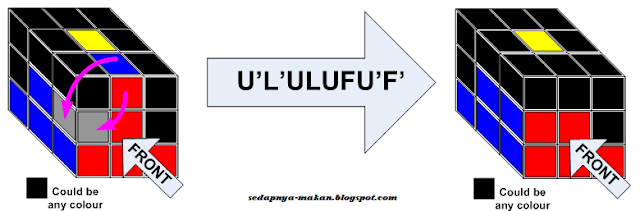Figure 33: using the algorithm U’L’ULUFU’F’

c) Case 3 (the cube is in the correct position but the color is incorrect)
If we find this case as shown in figure 34, we have to remove this cube from that position. After that, we need to apply the algorithm to case 1 or case 2 to solve the problem. The algorithm to remove this cube from that position is shown in figure 35. Note that, this algorithm is only to remove the cube. The position cube is correct but the colors are in reverse condition.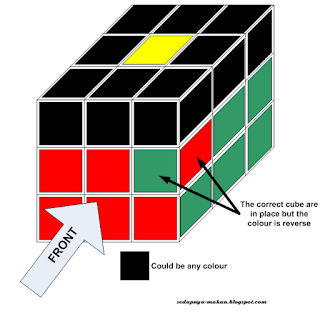Figure 34: the Correct cube is in place but the colors are reversed.
ghghgFigure 35: using the algorithm URU’R’U’F’UF

_________________________________________________________________________

STEP 4 (solving pattern 4)
?
?

STEP 5 (solving pattern 5)
?
?

STEP 6 (solving pattern 6)
?
?

STEP 7 (solving pattern 7)
?
?

If you want to know the remaining step to complete the task, you can find the all the steps in the book as below:

or

CONCLUSION
Now you know how to solve the trick of Rubik's cube. These easy 7 steps can help you to be more understood about the Rubik's cube. You can play around in front of your friend to get their attention. Make your friend impress with you. Good luck!!!!